# Chip Model for Addition and Subtraction

About 32 years ago I had just completed my B.S. in Mathematics and was immediately hired to teach mathematics at Roosevelt Junior High School in Oakland, California. I thought I knew everything there was to know about K-12 math concepts. Most certainly, adding and subtracting whole numbers!

However, I was almost immediately humbled by a student’s innocent question in the newcomers class I was teaching. This was a class made up of students who were new to the United States, and therefore the typical student in this class had little or no prior educational experience in the country they came from.

“Why do you have to cross off that top number and put a little one over there?”, she said, pointing to the subtraction problem I had just expertly solved on the chalkboard.

I gave some sort of lame answer about the top number not having enough, so he goes next door to borrow a one…blah…blah…blah. It was at that moment that I knew it was not enough to know the algorithms, but I had know WHY they worked and how to explain them to students.

I have come to love the CHIP MODEL for visually representing the four operations of whole numbers because it makes sense and it directly parallels the standard algorithm.

Modeling numbers

Before we model the operations, let’s first begin by using the chip model to visually represent numbers.

 268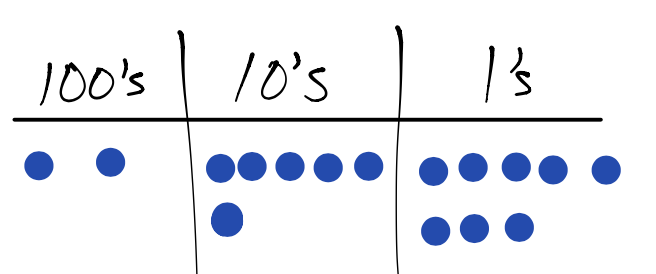326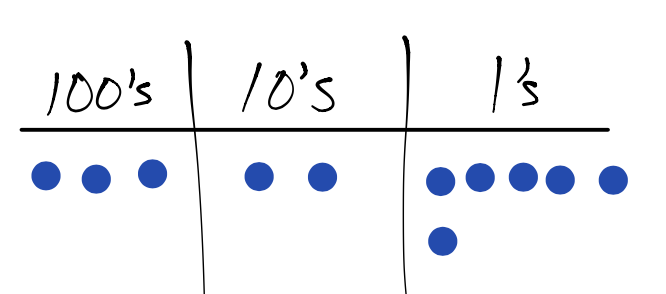The chips are a visual representation of the place value system in mathematics.

Exchange rate

Because we use the base-ten system, we can exchange 10 dots in one column for 1 dot in the column to the left. If this seems confusing, let’s use base-ten blocks to take a look at the same “exchange” idea.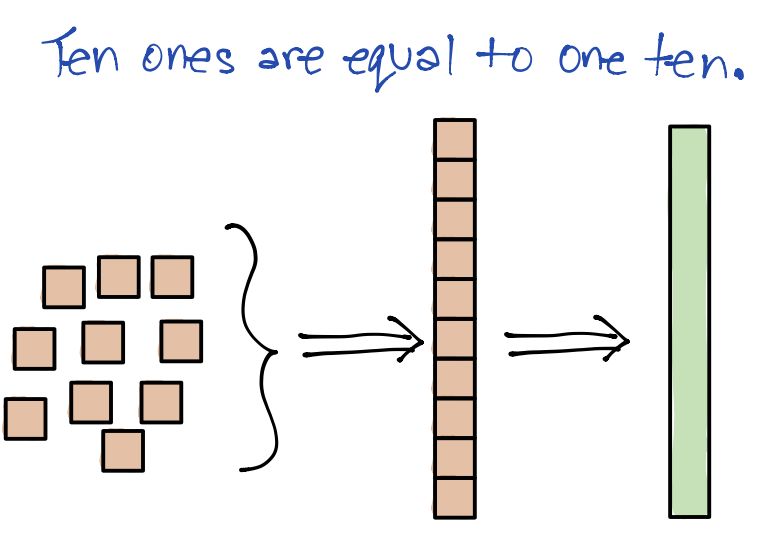Ten unit cubes can be lined up to equal a single ten-rod.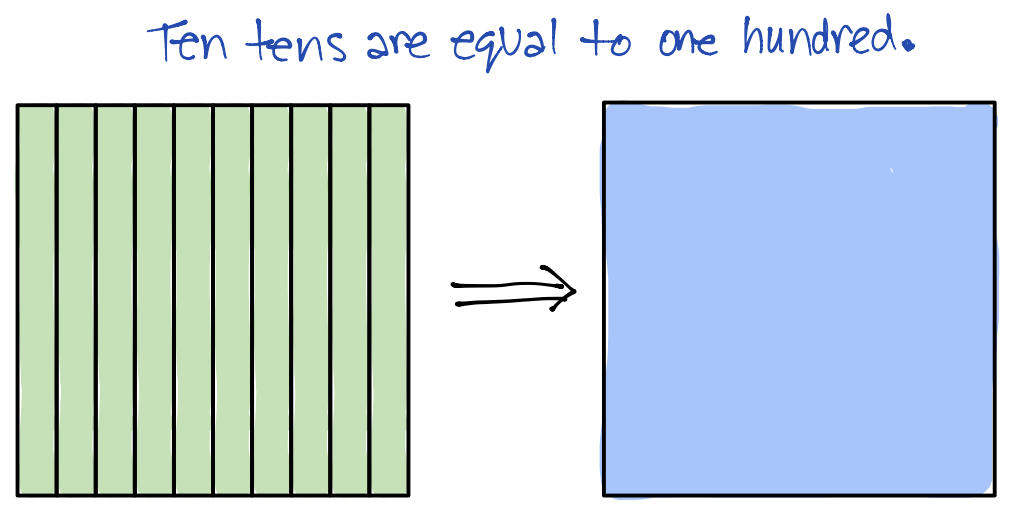Similarly, 10 ten-rods can be arranged to equal a single hundred-square.

These two exchanges can be generalized into a single generalized statement: ten “littles” equals one “big”

Using base-ten blocks are using for introducing this exchange rate because the relative size of the blocks are proportional to one another. Studnets can literally count that ten unit cubes equals 1 ten-rod and 10 ten-rods is the same size as a single hundred-square.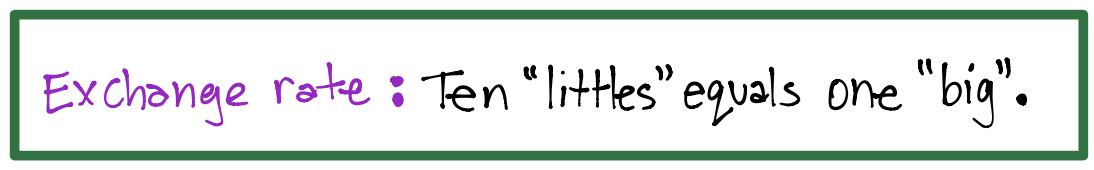There is no need to move on to the chip model until students truly understand the concept of exchange rate.

Exchange rate with chips

The exchange rate becomes relevant when we want to express a number in its simplest form.

What is the value of 3 hundreds, 2 tens, and 14 ones?

After modeling the values, we see that there is one opportunity to do an exchange in the one’s column. Since there are 14 ones, we can exchange ten of the ones for a single dot in the ten’s column.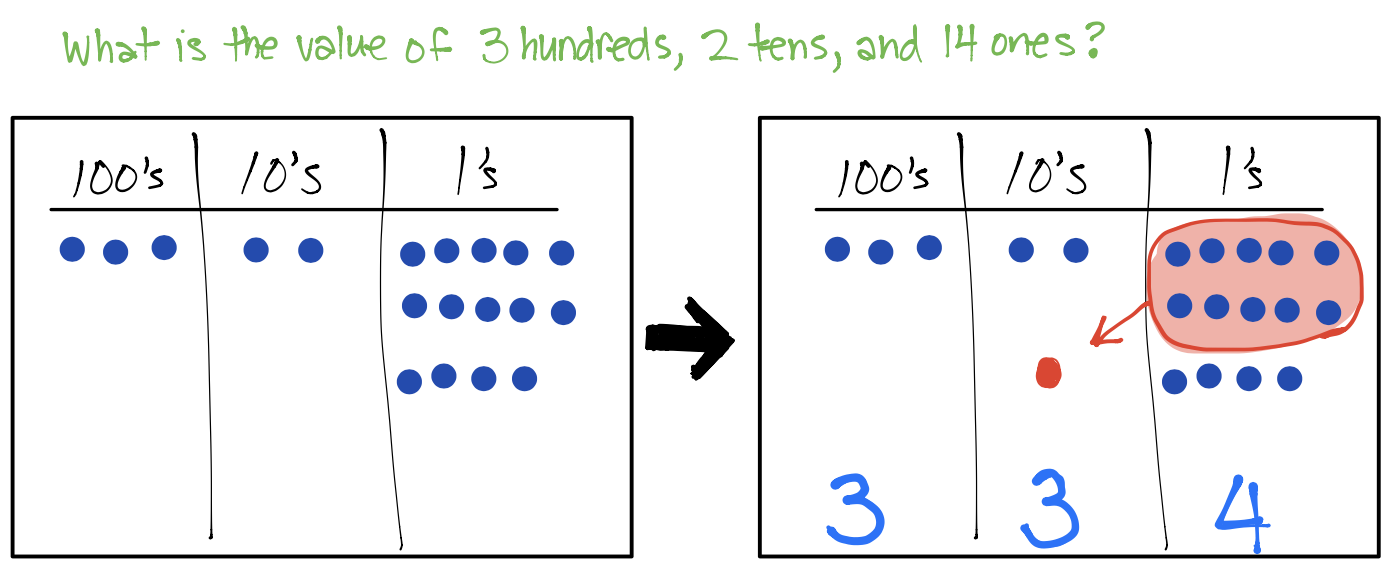Here is another example, this time required two exchanges.

What is the value of 3 hundreds, 16 tens, and 13 ones?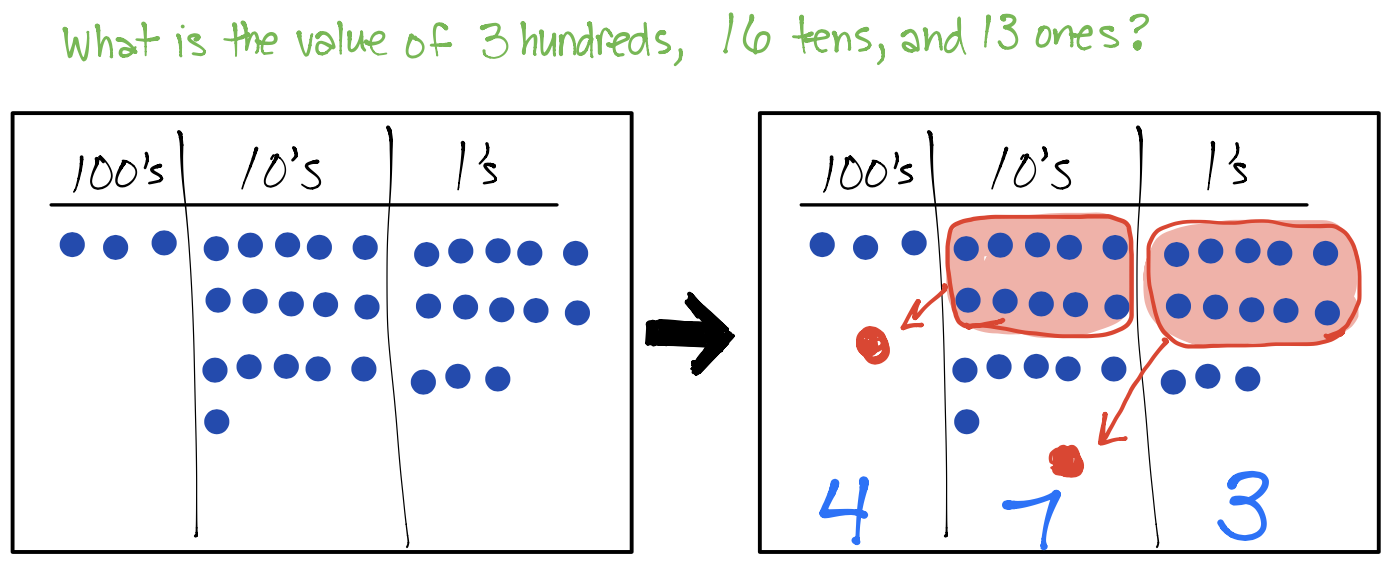Suppose we want to add 268 and 147 using the chip model.

First we define addition as combining things. Adding 268 and 147 means we will be combining the dots representing 268 with the dots representing 147.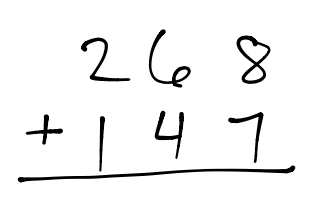Begin by modeling both numbers on the same place value chart. At this point, we can see that the unsimplified sum is 3 hundreds and 10 tens and 15 ones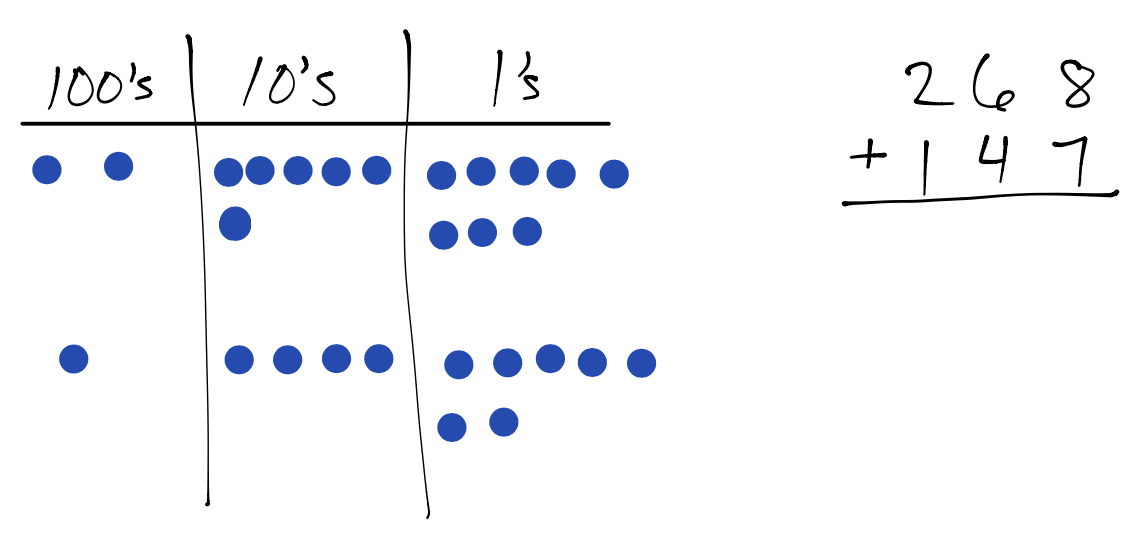Now we look for opportunities to do the exchange rate of 10 littles equals 1 big. We see there is a two opportunities to do so because there are 10 dots in the tens column and 15 dots in the ones column. It does not matter which exchange we do first, so we will begin by exchanging 10 ones for 1 ten.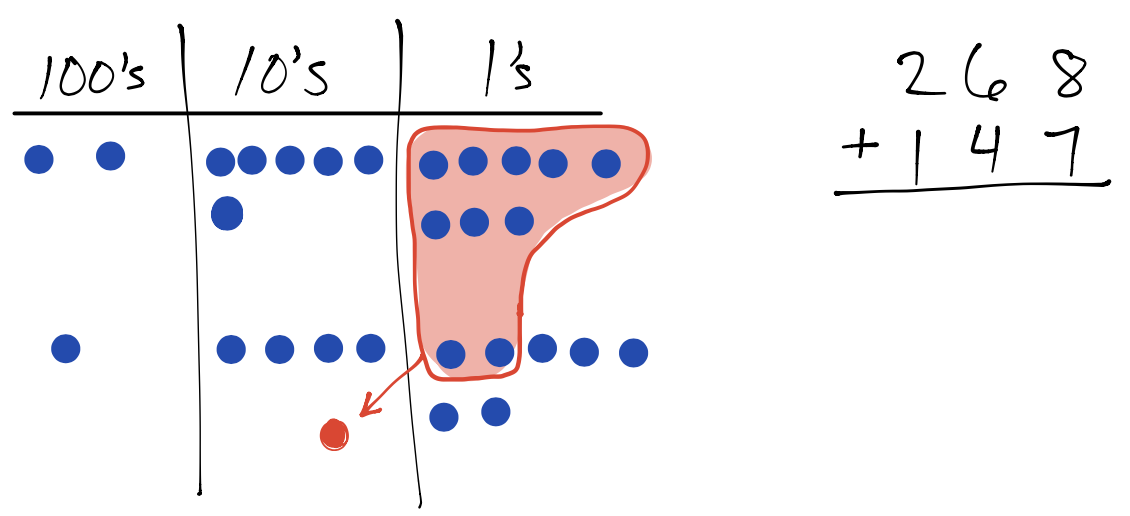Now we can do the remaining exchange of 10 tens for 1 hundred.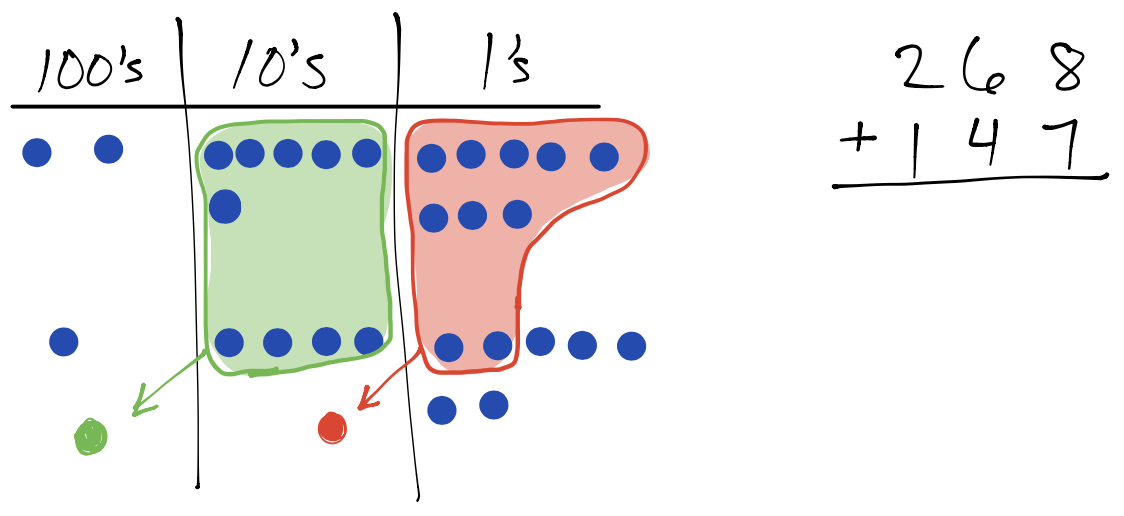There are no additional exchanges that can be made, so we count the remaining dots in each column. 4 hundreds and 1 ten and 5 ones The sum is 415.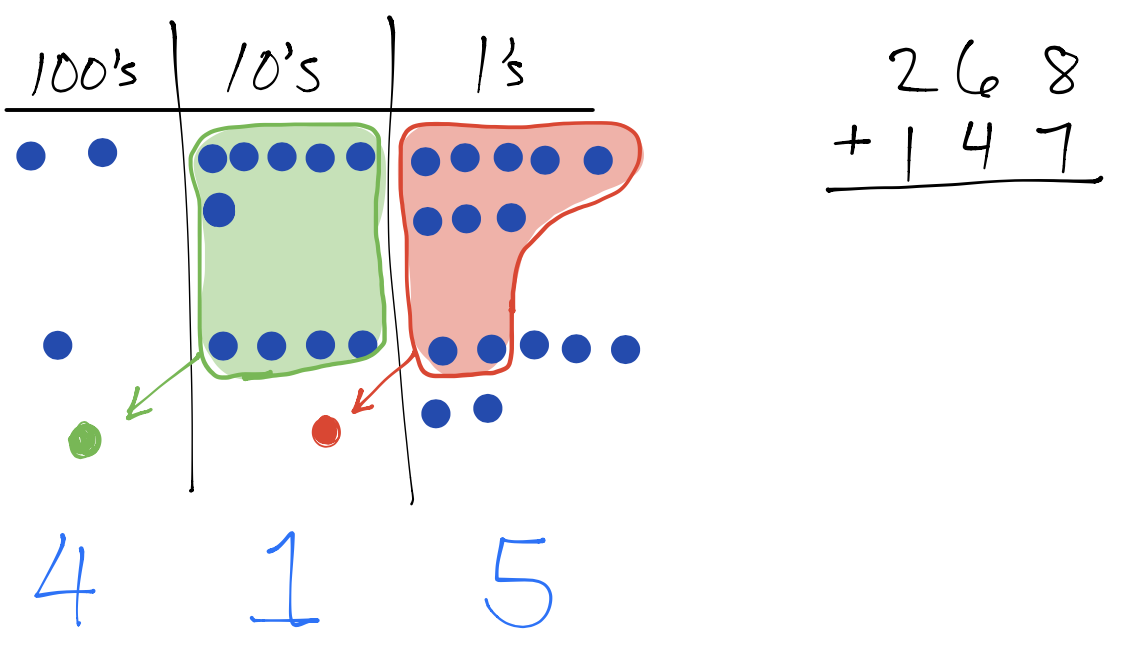Notice that all the work students would be doing is with the dots. We haven’t actually recorded any of the steps in the standard algorithm. This is by design! We need to give students plenty of experience with the visual model to make sense of the math concept BEFORE moving to the more abstract algorithm.

Eventually, students will show the chip model and the algorithm side-by-side, using the chip model one the left and recording each step with numbers on the right side.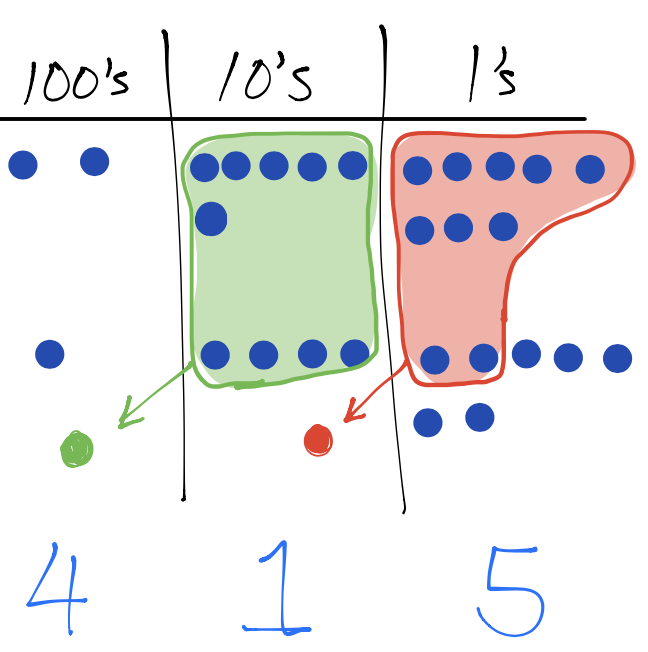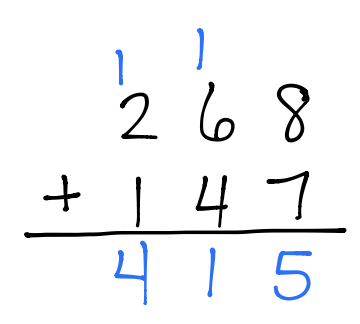In a nutshell, there are only two components of addition that we need students to understand:

1. Addition means to combine things
2. The exchange rate is 10 littles equals 1 big

Now we just need to provide our students with numerous opportunities to practice. During their practice, students will naturally seek strategies for becoming more efficient.

Subtraction with the chip model

As mentioned earlier, we want to explain subtraction with regrouping in a way that is mathematically sound rather than resorting to mnemonics and metaphors. We can achieve this by using our understanding of addition to make sense of subtraction.

The two components of addition leads us to the two components of subtraction:

1. Subtract means to take away things
2. The exchange rate is 1 big equals 10 littles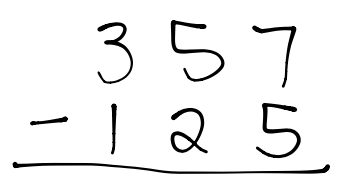The expression 357 – 125 means we take away 1 hundred and 2 tens and 5 ones from the dots that represent 357.

 Begin by modeling 357 on the place value chart. We do not need to model 125 because 125 represents the amount we need to remove from the place value chart.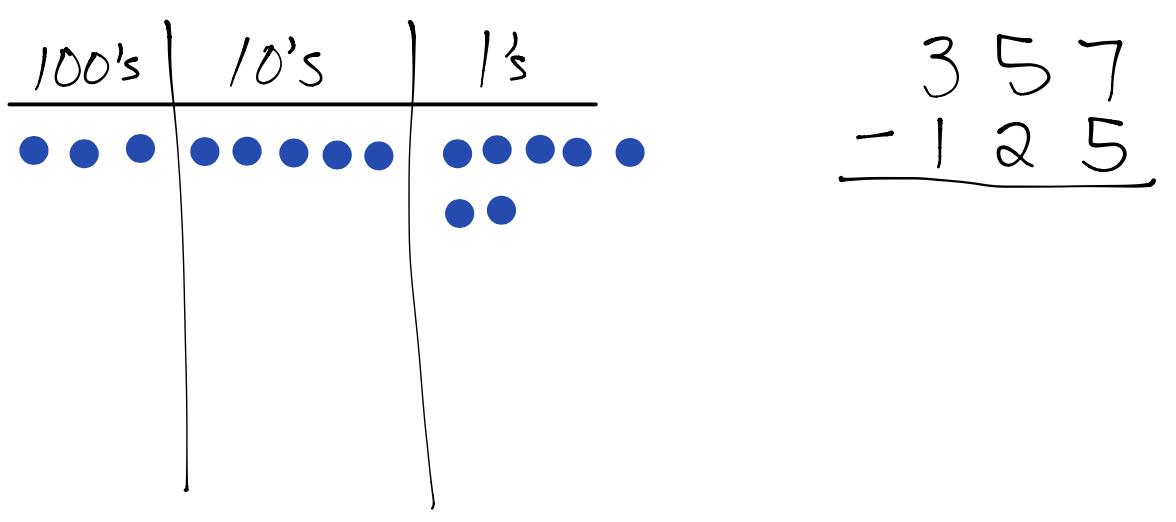Model the subtraction by crossing off 1 hundred and 2 tens and 5 ones from the dots.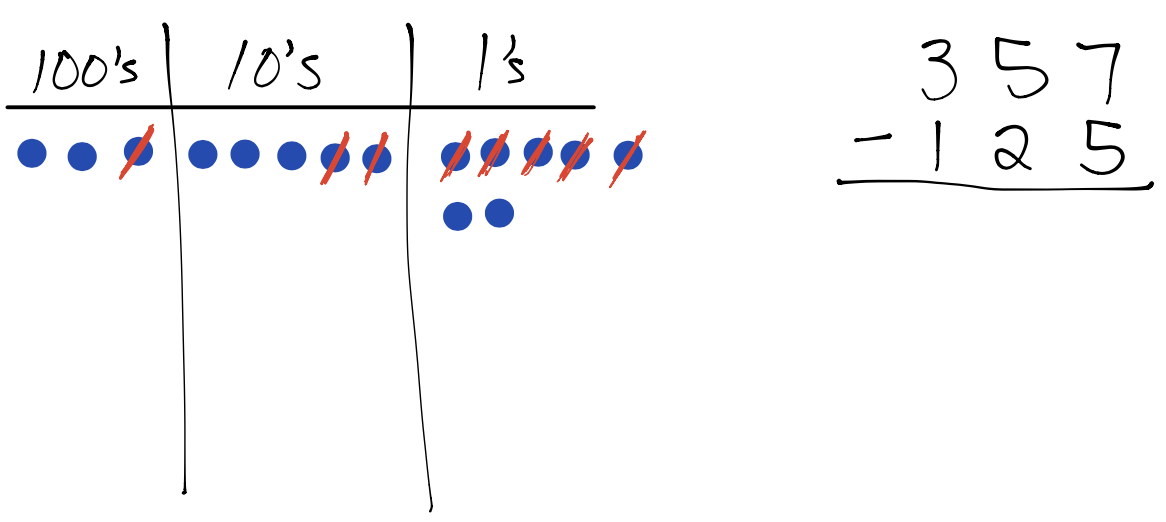The remaining dots represent the difference. 2 hundreds and 3 tens and 2 ones …or more simply… 232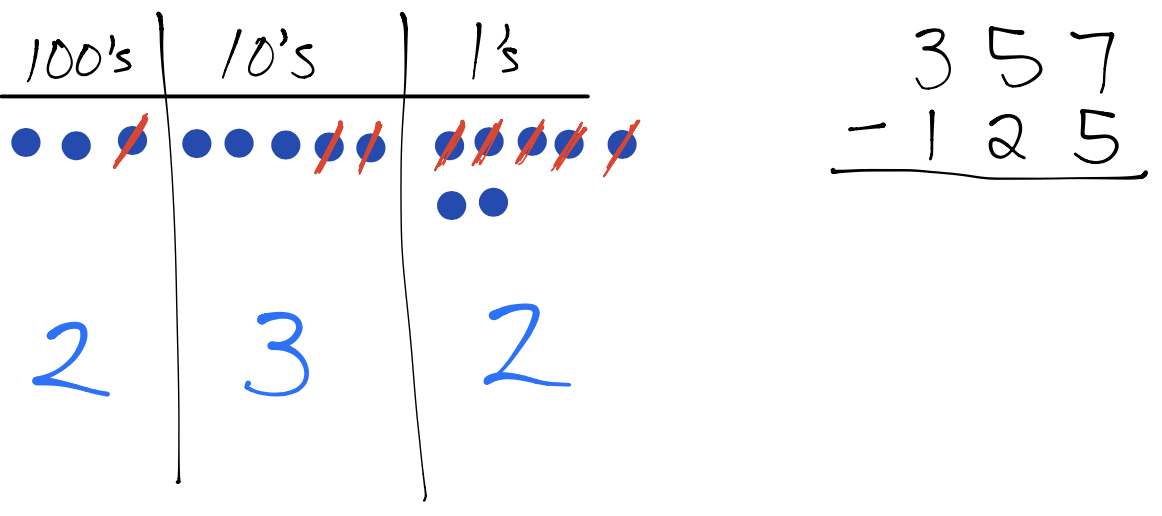That previous example did not require regrouping, so here is one that does require it.

326 – 159

 Begin by modeling 326 on the place value chart. Technically, we can subtract left-to-right or right-to-left, but for our purposes we will go right-to-left in order to model the standard algorithm in the United States.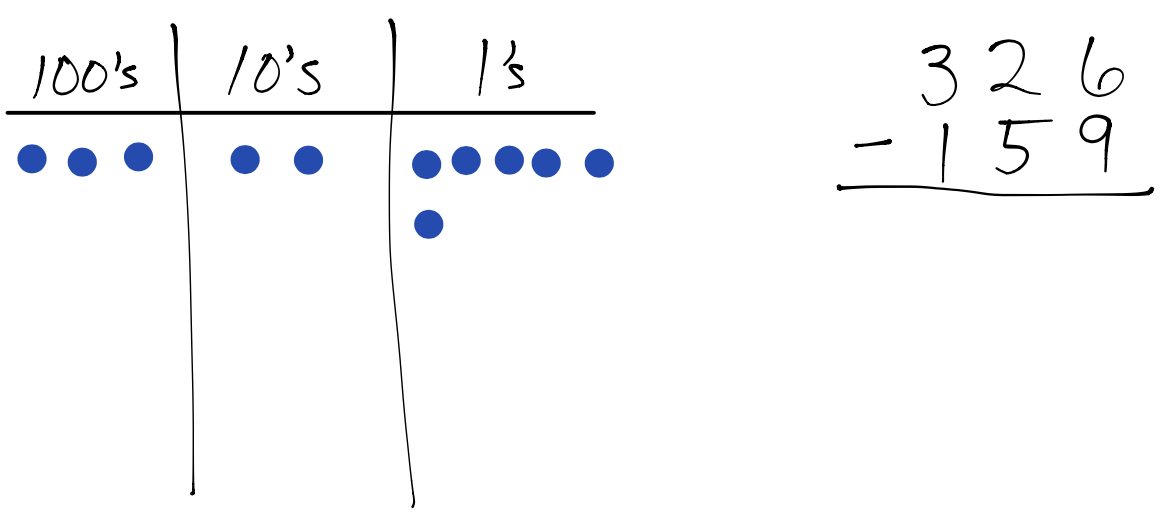We need to remove 9 ones, but our model only has 6 ones showing, so we use the exchange rate of 1 big equals 10 littles which now gives us 16 ones.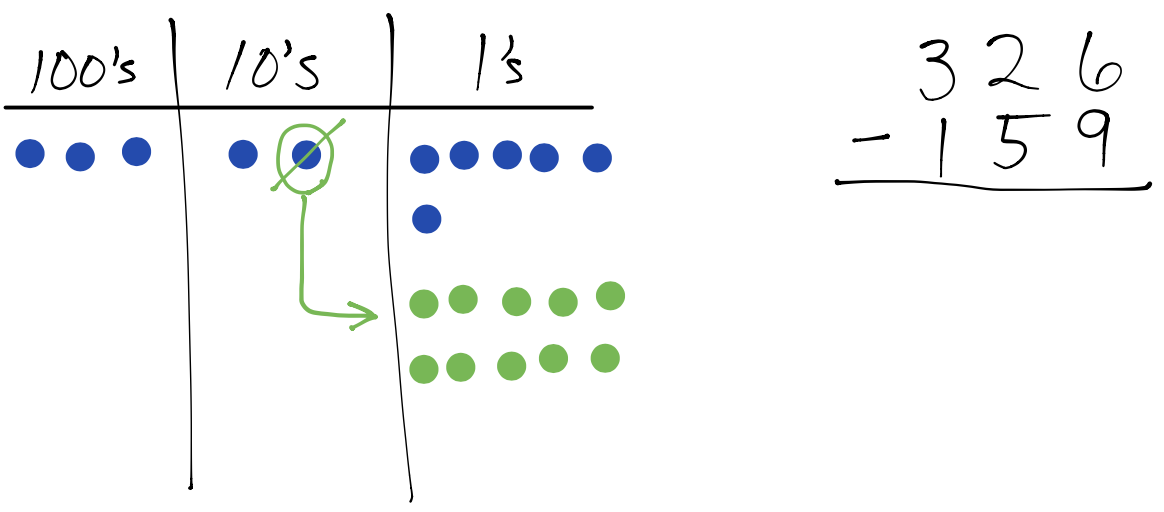Now we can cross off 9 ones. We use a ✔ to indicate that we have crossed off the 9 ones and are ready to move to the tens column.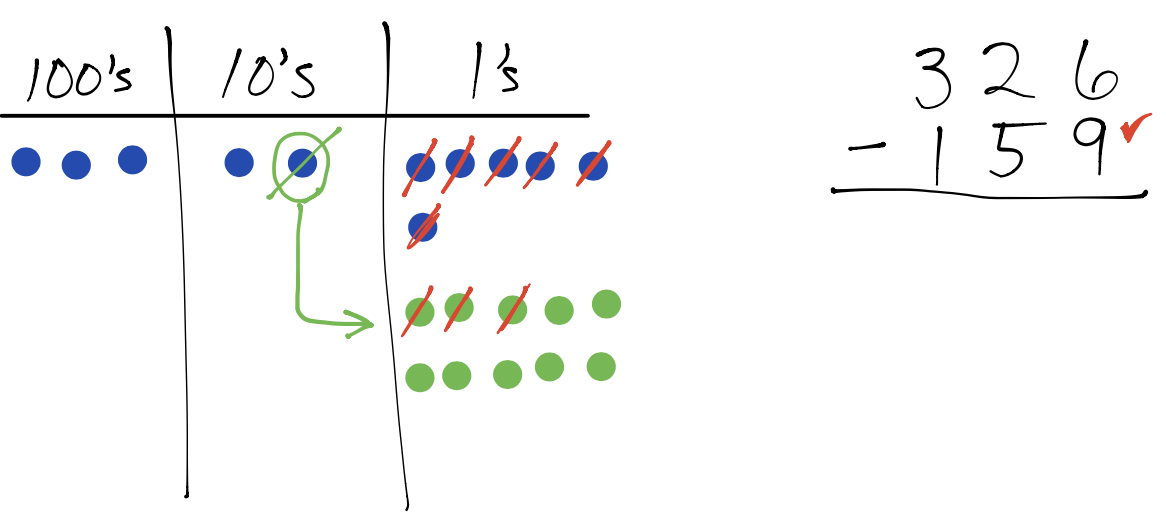Now we need to cross off 5 tens, but we only currently have 1 ten, so we use the exchange rate of 1 big equals 10 littles which now gives us 11 tens.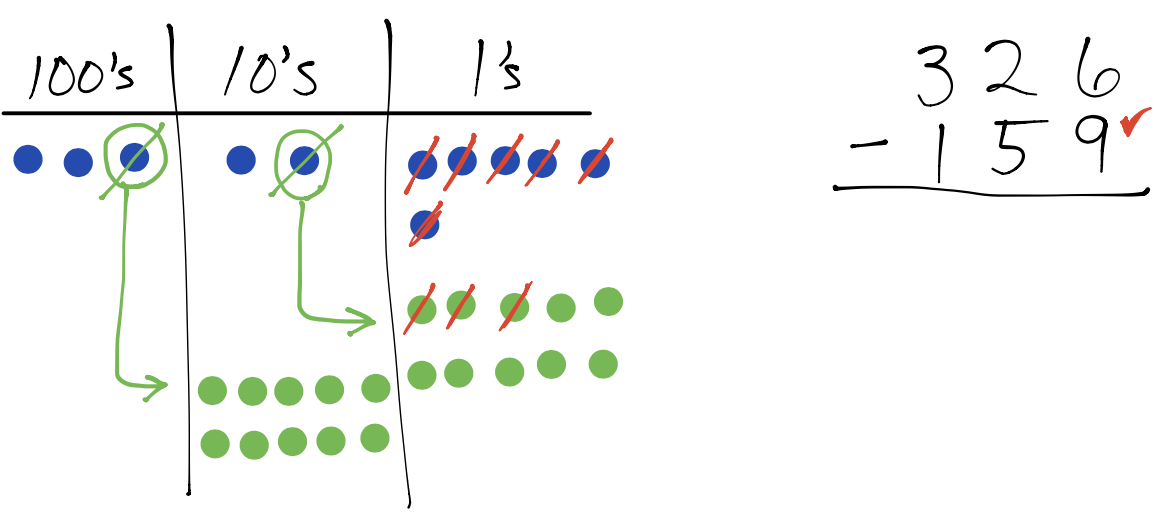Now we can cross off 5 tens. We use a ✔ to indicate that we have crossed off the 5 tens and are ready to move to the hundreds column.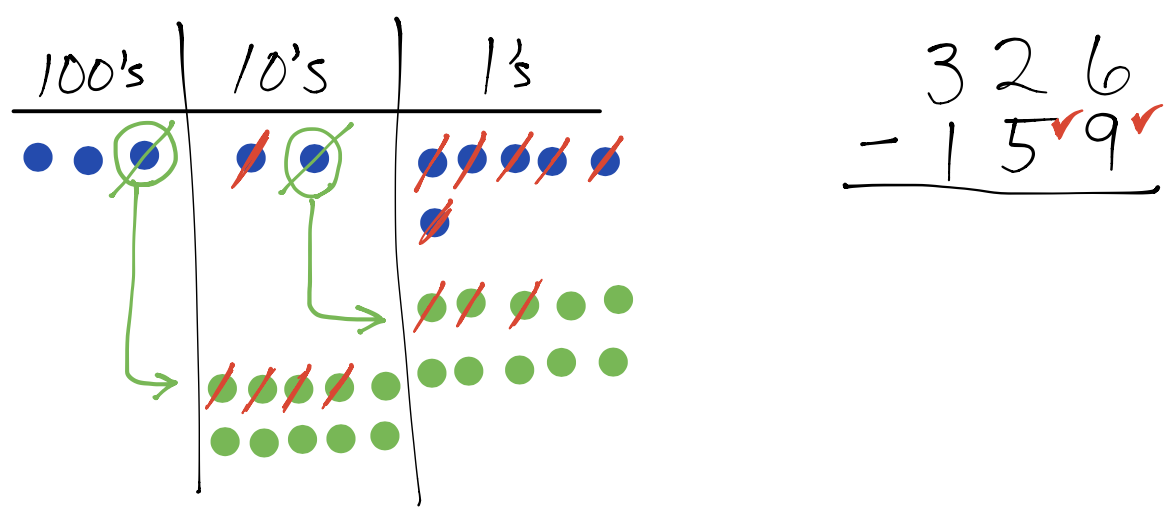Our final step is to cross off 1 hundred, which we can do because we have 2 hundreds remaining in the hundreds column. A ✔ in the hundreds column indicates we are finished removing 1 hundred, 5 tens, and 9 ones.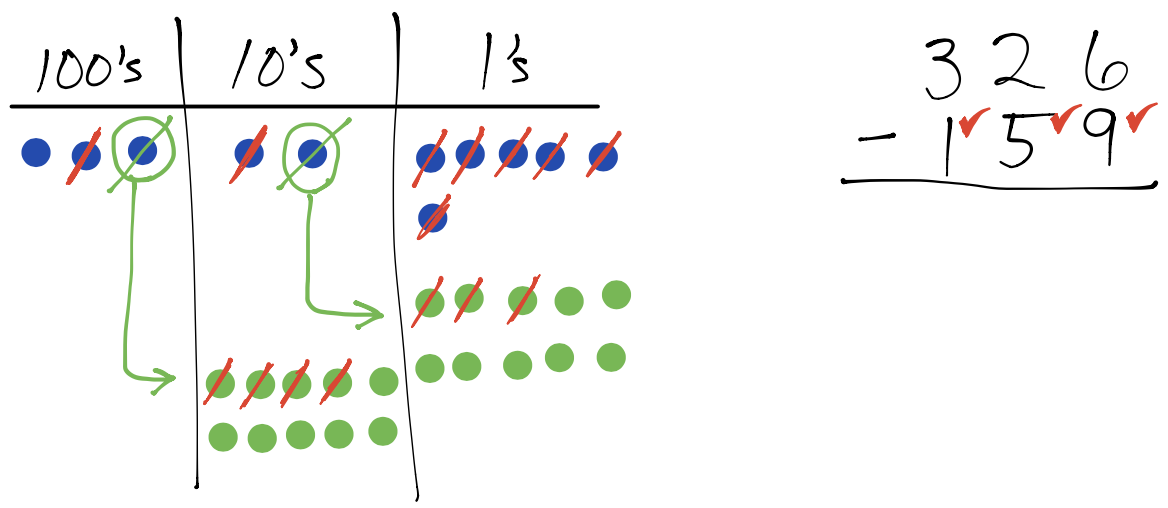The remaining dots represent the difference.  1 hundred and 6 tens and 7 ones …or more simply… 167Using the chip model, we condense all the addition and subtraction lessons from 1st grade through 4th grade into three simple truths:

1. The exchange rate is always 10 littles equals 1 big (and its corollary that 1 big equals 10 littles)
2. Addition means to combine things
3. Subtraction means to remove things

Special cases like adding and subtracting across columns of zeros are merely natural consequence of the three truths and no longer cause students to think they have to learn how to handle a bunch of “what if” scenarios.

 Addition across zeros Subtraction across zeros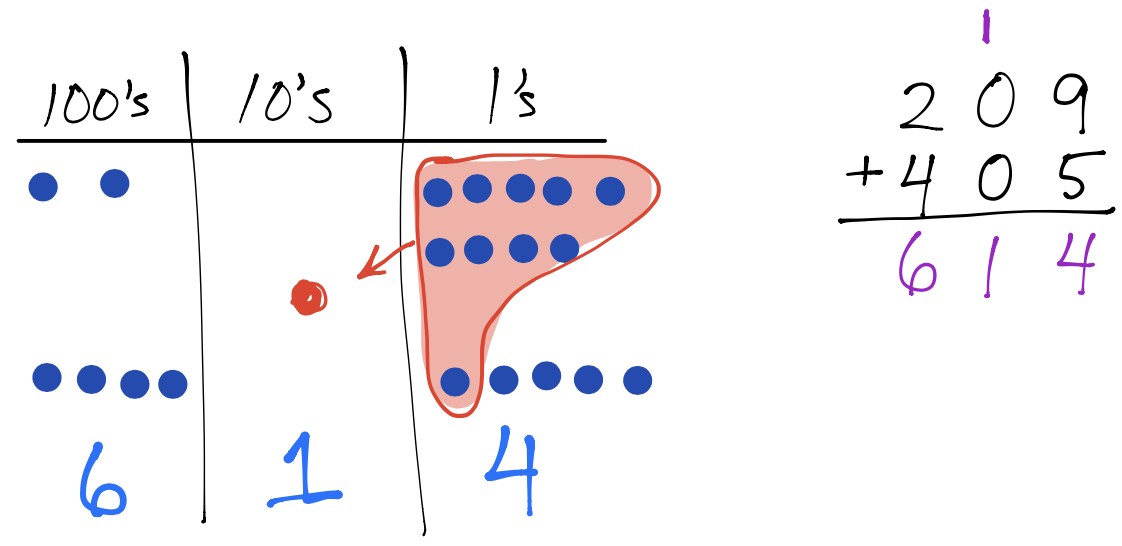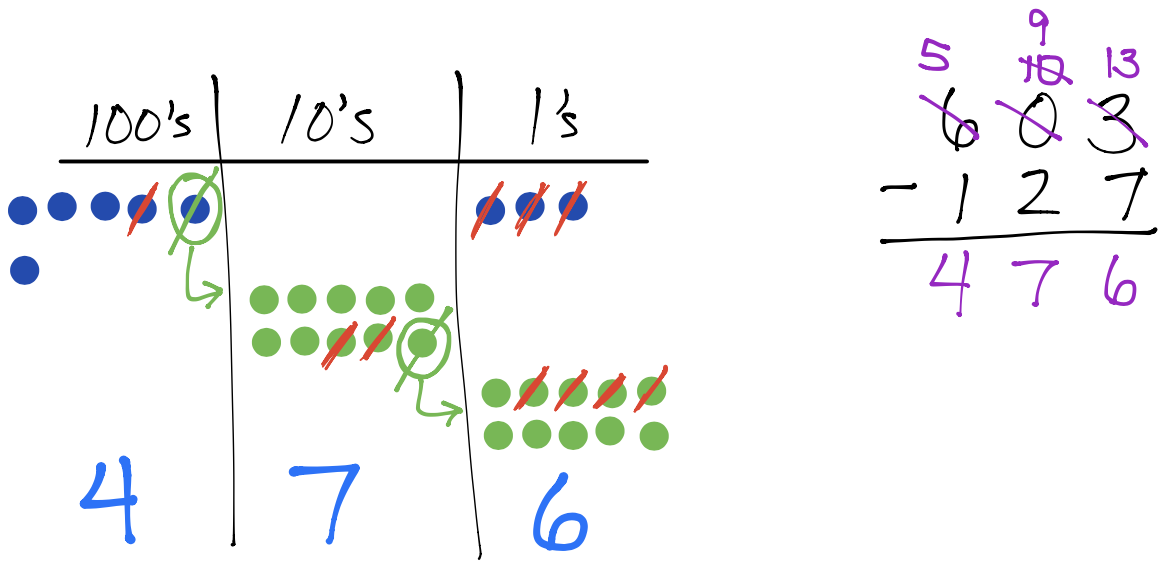Multiplication in the next post! You can view it here.

.

.

.